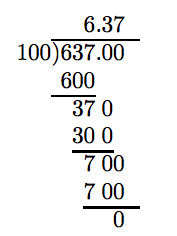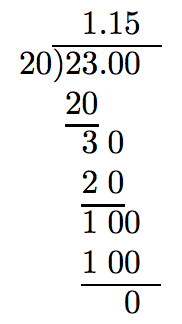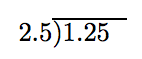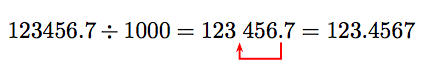# 3.4.2: Dividing Decimals

$$\newcommand{\vecs}{\overset { \rightharpoonup} {\mathbf{#1}} }$$ $$\newcommand{\vecd}{\overset{-\!-\!\rightharpoonup}{\vphantom{a}\smash {#1}}}$$$$\newcommand{\id}{\mathrm{id}}$$ $$\newcommand{\Span}{\mathrm{span}}$$ $$\newcommand{\kernel}{\mathrm{null}\,}$$ $$\newcommand{\range}{\mathrm{range}\,}$$ $$\newcommand{\RealPart}{\mathrm{Re}}$$ $$\newcommand{\ImaginaryPart}{\mathrm{Im}}$$ $$\newcommand{\Argument}{\mathrm{Arg}}$$ $$\newcommand{\norm}{\| #1 \|}$$ $$\newcommand{\inner}{\langle #1, #2 \rangle}$$ $$\newcommand{\Span}{\mathrm{span}}$$ $$\newcommand{\id}{\mathrm{id}}$$ $$\newcommand{\Span}{\mathrm{span}}$$ $$\newcommand{\kernel}{\mathrm{null}\,}$$ $$\newcommand{\range}{\mathrm{range}\,}$$ $$\newcommand{\RealPart}{\mathrm{Re}}$$ $$\newcommand{\ImaginaryPart}{\mathrm{Im}}$$ $$\newcommand{\Argument}{\mathrm{Arg}}$$ $$\newcommand{\norm}{\| #1 \|}$$ $$\newcommand{\inner}{\langle #1, #2 \rangle}$$ $$\newcommand{\Span}{\mathrm{span}}$$$$\newcommand{\AA}{\unicode[.8,0]{x212B}}$$

In this and following sections we make use of the terms divisor, dividend, quotient, and remainder.

Divisor, Dividend, Quotient, and Remainder

This schematic reminds readers of the position of these terms in the division process.Now that these terms are defined, we begin the discussion of division of decimal numbers. Suppose that we wish to divide 637 by 100. We could do this in fraction form, change the result to a mixed fraction, then the mixed fraction to decimal form.

$\frac{637}{100} = 6 \frac{37}{100} = 6.37\nonumber$

We can also arrange the division much as we would the division of two whole numbers.Note that adding two zeros after the decimal point in the dividend does not change the value of 637. Further, note that we proceed as if we are dividing two whole numbers, placing the decimal point in the quotient directly above the decimal point in the dividend.

These observations lead to the following algorithm.

Dividing a Decimal by a Whole Number

To divide a decimal number by a whole number, proceed as follows:

1. Set up the long division as you would the division of two whole numbers.
2. Perform the division as if the numbers were both whole numbers, adding zeros to the right of the decimal point in the dividend as necessary to complete the division.
3. Place the decimal point in the quotient immediately above the decimal point in the dividend.

Example 1

Divide 23 by 20.

Solution

Arrange as if using long division to divide whole numbers, adding enough zeros to the right of the decimal point in the dividend to complete the division.Hence, 23 divided by 20 is 1.15.

Adding Zeroes to the Right of the Decimal Point

Usually, one does not immediately see how many zeros to the right of the decimal point in the dividend are needed. These zeros are usually added at each step of the division, until the division is completed or the user is willing to terminate the process and accept only an estimate of the quotient.

Example 2

Divide: 155.2 ÷ 25.

Solution

Arrange as if using long division to divide whole numbers, and begin.We still have a nonzero remainder. Adding another zero does no good.However, if we add one more additional zero, the division completes with a zero remainder.Thus, 155.2 divided by 25 is 6.208.

Exercise

Divide: 42.55 ÷ 23

1.85

Decimal Divisors When the divisor contains a decimal point, we have a little work to do before we begin the division process. Suppose that we wish to divide 1.25 by 2.5. In fraction form, we could start with

$\frac{1.25}{2.5},\nonumber$

then clear the decimals from the denominator by multiplying both numerator and denominator by 10. Note: Recall that multiplying by 10 moves the decimal point one place to the right.

\begin{aligned} \frac{1.25}{2.5} = \frac{1.25 \cdot 10}{2.5 \cdot 10} \\ = \frac{12.5}{25} \end{aligned}\nonumber

Thus, dividing 1.25 by 2.5 is equivalent to dividing 12.5 by 25. This we know how to do.Thus, 1.25 divided by 2.5 is 0.5.

Writing Mathematics

Never write .5. Always add the leading zero in the ones place and write 0.5.

Instead of working in fraction form, we can take care of positioning the decimal point in the long division framework. Start with:Move the decimal point in the divisor to the end of the divisor, then move the decimal point in the dividend an equal number of places.Thus, the division becomesand we proceed as above to find the quotient. This discussion motivates the following algorithm.

Dividing by a Decimal Divisor

If the divisor contains a decimal, proceed as follows:

1. Move the decimal to the end of the divisor.
2. Move the decimal in the dividend an equal number of places.

We can then complete the division using the rules for dividing a decimal by a whole number.

Example 3

Divide: $$\overline{ )4.392}$$

Solution

Move the decimal in the divisor to the end of the divisor. Move the decimal in the dividend an equal number of places (two places) to the right.Now we can follow the algorithm for dividing a decimal number by a whole number.Thus, 4.392 divided by 0.36 is 12.2.

Exercise

Divide: $$0.45 \overline{)36.99}$$

82.2

## Dividing Signed Decimal Numbers

The rules governing division of signed decimal numbers are identical to the rules governing division of integers.

Like Signs. The quotient of two decimal numbers with like signs is positive. That is:

$\frac{(+)}{(+)} = + \text{ and } \frac{(−)}{(−)} = +\nonumber$

Unlike Signs. The quotient of two decimal numbers with unlike signs is negative. That is:

$\frac{(+)}{(−)} = − \text{ and } \frac{(−)}{(+)} = −\nonumber$

Example 4

Divide: −0.03 ÷ 0.024.

Solution

First, divide the magnitudes. Move the decimal in the divisor to the end of the divisor. Move the decimal in the dividend an equal number of places (three places) to the right. Note that this requires an extra trailing zero in the dividend.Our problem then becomes:

$24 \overline{24 )30}\) We can now follow the algorithm for dividing a decimal number by a whole number. Note that we have to add two trailing zeros in the dividend to complete the division with a zero remainder.Finally, because the quotient of unlike signs is negative, −0.03 divided by 0.024 is −1.25. That is, \[ \frac{-0.03}{0.024} = -1.25.\nonumber$

Exercise

Divide: −0.0113 ÷ 0.05

−0.226

## Rounding

Sometimes an exact decimal representation of a fraction is not needed and is an approximation is more than adequate.

Example 5

Convert 4/7 to a decimal. Round your answer to the nearest hundredth.

Solution

We need to carry the division one place beyond the hundredths place.To round to the nearest hundredth, first identify the rounding and test digits.Because the “test digit” is less 5, leave the rounding digit alone and truncate. Therefore, correct to the nearest hundredth, 4/7 ≈ 0.57.

Exercise

Convert 5/7 to a decimal. Round your answer to the nearest hundredth.

0.71

## Dividing by Powers of Ten

Recall:

\begin{aligned} 10^1 = 10 \\ 10^2 = 10 \cdot 10 = 100 \\ 10^3 = 10 \cdot 10 \cdot 10 = 1000 \end{aligned}\nonumber

Powers of Ten

In the expression 10n, the exponent matches the number of zeros in the answer. Hence, 10n will be a 1 followed by n zeros.

Thus, 104 = 10, 000, 105 = 100, 000, etc. The exponent tells us how many zeros will follow the 1.

Let’s divide 123456.7 by 1000.Note the result: 123456.7 divided by 1000 is 123.4567. Dividing by 1000 moves the decimal point 3 places to the left!This discussion leads to the following result.

Dividing a Decimal by a Power of Ten

Dividing a decimal number by 10n will move the decimal point n places to the left.

Example 6

Simplify: 123456.7 ÷ 104

Solution

Dividing by 104 (or equivalently, 10,000) moves the decimal point four places to the left. Thus, 123456.7 ÷ 104 = 12.34567.

Exercise

Simplify: 123456.7 ÷ 102

1234.567

## Order of Operations

We remind readers of the Rules Guiding Order of Operations.

Rules Guiding Order of Operations

When evaluating expressions, proceed in the following order.

1. Evaluate expressions contained in grouping symbols first. If grouping symbols are nested, evaluate the expression in the innermost pair of grouping symbols first.
2. Evaluate all exponents that appear in the expression.
3. Perform all multiplications and divisions in the order that they appear in the expression, moving left to right.
4. Perform all additions and subtractions in the order that they appear in the expression, moving left to right.

In addition, when fractions are present:

Fractional Expressions

If a fractional expression is present, simplify the numerator and denominator separately, then divide.

Example 7

Evaluate ab/(c + d), given that a = 2.1, b = −3.4, c = −1.3, and d = 1.1.

Solution

Recall that it is good practice to prepare an expression for substitution by using parentheses.

$ab/(c + d) = (~)(~)/((~) +(~)) \nonumber$

Substitute the given values for a, b, c, and d, then use the Rules Guiding Order of Operations to simplify the resulting expression.

\begin{aligned} ab/(c+d) = (2.1)(-3.4)/((-1.3)+(1.1)) ~ & \textcolor{red}{2.1, -3.4,-1.3, 1.1 \text{ for } a, b, c, d.} \\ = (2.1)(-3.4)/(-0.2) ~ & \textcolor{red}{ \text{ Parens: } (-1.3) + (1.1) = -0.2.} \\ =-7.14/(-0.2) ~ & \textcolor{red}{ \text{ Multiply: } (2.1)(-3.4) = -7.14.} \\ =35.7 ~ & \textcolor{red}{ \text{ Divide: } -7.14/(-0.2) = 35.7} \end{aligned}\nonumber

Exercise

If a = −2.1, b = 1.7, c = 4, and d = 0.05, evaluate:

$\frac{a+b}{cd}\nonumber$

−2

Example 8

Given a = 0.1 and b = −0.3, evaluate the expression

$\frac{a+2b}{2a+b}.\nonumber$

Solution

Substitute the given values, then use the Rules Guiding Order of Operations to simplify the resulting expression.

\begin{aligned} \frac{a+2b}{2a+b} = \frac{(0.1)+2(-0.3)}{2(0.1)+(-0.3)} ~ & \textcolor{red}{ \text{ 0.1 for } a,~ -0.3 \text{ for } b.} \end{aligned}\nonumber

Simplify the numerator, simplify the denominator, then divide.

\begin{aligned} = \frac{0.1+(-0.6)}{0.2+(-0.3)} ~ & \textcolor{red}{ \begin{aligned} \text{ Numerator: } 2(-0.3) = -0.6. \\ \text{ Denominator: } 2(0.1)=0.2. \end{aligned}} \\ = \frac{-0.5}{-0.1} ~ & \textcolor{red}{ \begin{aligned} \text{ Numerator: } 0.1 + (-0.6)=-0.5 \\ \text{ Denominator: } 0.2 + (-0.3) = -0.1. \end{aligned}} \\ =5 ~ & \textcolor{red}{ \text{ Divide: } -0.5/(-0.1)=5.} \end{aligned}\nonumber

Exercise

If a = 0.5 and b = −0.125, evaluate:

$\frac{2a-b}{a+2b}\nonumber$

4.5

## Exercises

In Exercises 1-16, divide the numbers.

1. $$\frac{39}{52}$$

2. $$\frac{16}{25}$$

3. $$\frac{755.3}{83}$$

4. $$\frac{410.4}{76}$$

5. $$\frac{333}{74}$$

6. $$\frac{117}{65}$$

7. $$\frac{32.12}{73}$$

8. $$\frac{12.32}{44}$$

9. $$\frac{37.63}{71}$$

10. $$\frac{20.46}{31}$$

11. $$\frac{138}{92}$$

12. $$\frac{110}{25}$$

13. $$\frac{17}{25}$$

14. $$\frac{18}{75}$$

15. $$\frac{229.5}{51}$$

16. $$\frac{525.6}{72}$$

In Exercises 17-40, divide the decimals.

17. $$\frac{0.3478}{0.47}$$

18. $$\frac{0.4559}{0.97}$$

19. $$\frac{1.694}{2.2}$$

20. $$\frac{1.008}{1.8}$$

21. $$\frac{43.61}{4.9}$$

22. $$\frac{22.78}{3.4}$$

23. $$\frac{1.107}{0.41}$$

24. $$\frac{2.465}{0.29}$$

25. $$\frac{2.958}{0.51}$$

26. $$\frac{5.141}{0.53}$$

27. $$\frac{71.76}{7.8}$$

28. $$\frac{14.08}{8.8}$$

29. $$\frac{0.8649}{0.93}$$

30. $$\frac{0.3901}{0.83}$$

31. $$\frac{0.6958}{0.71}$$

32. $$\frac{0.1829}{0.31}$$

33. $$\frac{1.248}{0.52}$$

34. $$\frac{6.375}{0.85}$$

35. $$\frac{62.56}{9.2}$$

36. $$\frac{28.08}{7.8}$$

37. $$\frac{6.278}{8.6}$$

38. $$\frac{3.185}{4.9}$$

39. $$\frac{2.698}{7.1}$$

40. $$\frac{4.959}{8.7}$$

In Exercises 41-64, divide the decimals.

41. $$\frac{−11.04}{1.6}$$

42. $$\frac{−31.27}{5.3}$$

43. $$\frac{−3.024}{5.6}$$

44. $$\frac{−3.498}{5.3}$$

45. $$\frac{−0.1056}{0.22}$$

46. $$\frac{−0.2952}{−0.72}$$

47. $$\frac{0.3204}{−0.89}$$

48. $$\frac{0.3306}{−0.38}$$

49. $$\frac{−1.419}{0.43}$$

50. $$\frac{−1.625}{−0.25}$$

51. $$\frac{−16.72}{−2.2}$$

52. $$\frac{−66.24}{9.2}$$

53. $$\frac{−2.088}{−0.87}$$

54. $$\frac{−2.025}{−0.75}$$

55. $$\frac{−1.634}{−8.6}$$

56. $$\frac{−3.094}{3.4}$$

57. $$\frac{−0.119}{0.85}$$

58. $$\frac{0.5766}{−0.62}$$

59. $$\frac{−3.591}{−6.3}$$

60. $$\frac{−3.016}{5.8}$$

61. $$\frac{36.96}{−4.4}$$

62. $$\frac{−78.26}{−8.6}$$

63. $$\frac{−2.156}{−0.98}$$

64. $$\frac{−6.072}{0.66}$$

In Exercises 65-76, divide the decimal by the given power of 10.

65. $$\frac{524.35}{100}$$

66. $$\frac{849.39}{100}$$

67. $$\frac{563.94}{10^3}$$

68. $$\frac{884.15}{10^3}$$

69. $$\frac{116.81}{10^2}$$

70. $$\frac{578.01}{10^3}$$

71. $$\frac{694.55}{10}$$

72. $$\frac{578.68}{100}$$

73. $$\frac{341.16}{10^3}$$

74. $$\frac{46.63}{10^4}$$

75. $$\frac{113.02}{1000}$$

76. $$\frac{520.77}{1000}$$

77. Compute the quotient 52/83, and round your answer to the nearest tenth.

78. Compute the quotient 43/82, and round your answer to the nearest tenth.

79. Compute the quotient 51/59, and round your answer to the nearest tenth.

80. Compute the quotient 17/69, and round your answer to the nearest tenth.

81. Compute the quotient 5/74, and round your answer to the nearest hundredth.

82. Compute the quotient 3/41, and round your answer to the nearest hundredth.

83. Compute the quotient 5/94, and round your answer to the nearest hundredth.

84. Compute the quotient 3/75, and round your answer to the nearest hundredth.

85. Compute the quotient 7/72, and round your answer to the nearest hundredth.

86. Compute the quotient 4/57, and round your answer to the nearest hundredth.

87. Compute the quotient 16/86, and round your answer to the nearest tenth.

88. Compute the quotient 21/38, and round your answer to the nearest tenth.

In Exercises 89-100, simplify the given expression.

89. $$\frac{7.5 \cdot 7.1 − 19.5}{0.54}$$

90. $$\frac{1.5(−8.8) − (−18.6)}{1.8}$$

91. $$\frac{17.76 − (−11.7)}{0.52}$$

92. $$\frac{−14.8 − 2.1}{2.62}$$

93. $$\frac{−18.22 − 6.7}{14.75 − 7.75}$$

94. $$\frac{1.4 − 13.25}{−6.84 − (−2.1)}$$

95. $$\frac{−12.9 − (−10.98)}{0.5^2}$$

96. $$\frac{5.1 − (−16.5)}{(−1.5)^2}$$

97. $$\frac{−9.5 \cdot 1.6 − 3.7}{−3.6}$$

98. $$\frac{6.5(−1.6) − 3.35}{−2.75}$$

99. $$\frac{−14.98 − 9.6}{17.99 − 19.99}$$

100. $$\frac{−5.6 − 7.5}{−5.05 − 1.5}$$

101. Given a = −2.21, c = 3.3, and d = 0.5, evaluate and simplify the following expression.

$\frac{a − c}{d^2}\nonumber$

102. Given a = 2.8, c = −14.68, and d = 0.5, evaluate and simplify the following expression.

$\frac{a − c}{d^2}\nonumber$

103. Given a = −5.8, b = 10.37, c = 4.8, and d = 5.64, evaluate and simplify the following expression:

$\frac{a − b}{c − d}\nonumber$

104. Given a = −10.79, b = 3.94, c = −3.2, and d = −8.11, evaluate and simplify the following expression:

$\frac{a − b}{c − d}\nonumber$

105. Given a = −1.5, b = 4.7, c = 18.8, and d = −11.75, evaluate and simplify the following expression.

$\frac{ab − c}{d}\nonumber$

106. Given a = 9.3, b = 6.6, c = 14.27, and d = 0.2, evaluate and simplify the following expression.

$\frac{ab − c}{d}\nonumber$

107. Biodiesel plants. There are about 180 biodiesel plants operating in about 40 states. Of the states that have them, what is the average number of biodiesel plants per state? Associated Press-Times-Standard 01/02/10 Fledgling biofuel industry ends year on a dour note.

108. Bat fungus. A fungus called ”white-nose syndrome” has killed an estimated 500,000 bats throughout the country. This means about 2,400,000 pounds of bugs aren’t eaten over the year, says Forest Service biologist Becky Ewing. How many pounds of insects does an average bat eat annually? Associated Press-Times-Standard 5/2/09

109. Patent backlog. In the U.S. Patent and Trademark Office, 6000 examiners have a backlog of 770,000 new, unexamined applications for patents. How many applications is that for each examiner to catch up on? Round your answer to the nearest tenth. Associated Press-Times-Standard 5/5/09

110. Doing well. The large health insurer Wellpoint, Inc., owner of Anthem Blue Cross, earned $536 million in the last three months of 2009. What was the average earnings per month for the insurer over that period? Round to the nearest million. Associated Press-Times-Standard 02/09/10 HHS secretary asks Anthem Blue Cross to justify rate hike. 111. Cyber attacks. The Pentagon has spent$100 million over a six-month period responding to and repairing damage from cyber-attacks and other computer network problems. What’s the average amount of money spent per month over that time? Round your answer to the nearest hundredth of a million. Associated Press-Times-Standard 4/19/09

112. Daily milk. The average California cow can produce 2,305 gallons of milk annually. How much milk can a cow produce each day? Round your answer to the nearest hundredth of a gallon. www.moomilk.com/faq.htm

113. Media mail. To promote her business, Theresa mails several packages via Media Mail. One package weighing 2 lbs. costs $2.77, another package weighing 3 lbs. costs$3.16, and the third package weighing 5 lbs. costs $3.94 to mail. What was the average cost per pound to mail the packages? Round your result to the nearest penny. www.usps.com/prices/media-mailprices.htm ## Answers 1. 0.75 3. 9.1 5. 4.5 7. 0.44 9. 0.53 11. 1.5 13. 0.68 15. 4.5 17. 0.74 19. 0.77 21. 8.9 23. 2.7 25. 5.8 27. 9.2 29. 0.93 31. 0.98 33. 2.4 35. 6.8 37. 0.73 39. 0.38 41. −6.9 43. −0.54 45. −0.48 47. −0.36 49. −3.3 51. 7.6 53. 2.4 55. 0.19 57. −0.14 59. 0.57 61. −8.4 63. 2.2 65. 5.2435 67. 0.56394 69. 1.1681 71. 69.455 73. 0.34116 75. 0.11302 77. 0.6 79. 0.9 81. 0.07 83. 0.05 85. 0.10 87. 0.2 89. 62.5 91. 117.84 93. −3.56 95. −7.68 97. 5.25 99. 12.29 101. −22.04 103. 19.25 105. 2.2 107. 4.5 biodiesel plants 109. 128.3 111.$16.67 million

113. \$0.99 per pound

This page titled 3.4.2: Dividing Decimals is shared under a CC BY-NC-SA license and was authored, remixed, and/or curated by David Arnold.